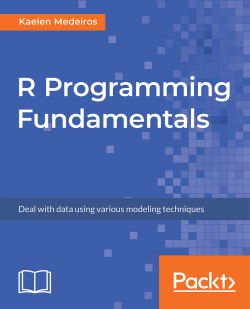# R Programming Fundamentals5 (1 reviews total)
By Kaelen Medeiros
• \$28.99 eBook
• \$36.99 Print + eBook
• \$12.99 eBook + Subscription
What do you get with a Packt Subscription?

• Constantly updated with 100+ new titles each month
• Breadth and depth in over 1,000+ technologies

R Programming Fundamentals, focused on R and the R ecosystem, introduces you to the tools for working with data. You’ll start by understanding how to set up R and RStudio, followed by exploring R packages, functions, data structures, control flow, and loops.

Once you have grasped the basics, you’ll move on to studying data visualization and graphics. You’ll learn how to build statistical and advanced plots using the powerful ggplot2 library. In addition to this, you’ll discover data management concepts such as factoring, pivoting, aggregating, merging, and dealing with missing values.

By the end of this book, you’ll have completed an entire data science project of your own for your portfolio or blog.

Publication date:
September 2018
Publisher
Packt
Pages
206
ISBN
9781789612998

# Introduction to R

One tool that statisticians—and now data scientists as well—often use for data cleaning, analysis, and reporting is the R programming language.

In this chapter, we'll begin by looking at the basics of using R as a programming language and as a statistical analysis tool, and we'll also install a few useful R packages that we will continue to use throughout the book.

By the end of this chapter, you will be able to:

• Install R packages for use throughout the book
• Use R as a calculator for basic arithmetic
• Utilize different data structures
• Control program flow by writing if-else, for, and while loops
• Import and export data to and from CSV, Excel, and SQL

# Using R and RStudio, and Installing Useful Packages

R is a programming language intended for use for statistical analysis. Additionally, it can be utilized in an object-oriented or functional way. Specifically, it is an implementation of S, an interactive statistical programming language. R was initially released in August 1993. It is maintained today by the R Development Core Team.

RStudio is an incredibly useful Integrated Development Environment (IDE) for writing and using R. Many data scientists use RStudio for writing R, as it provides a console window, a code editor, tools to help create plots and graphics, and can even be integrated with GitHub to support version control.

While R does share some functionality with Microsoft Excel, it allows you to have more control over your data, and you can add on a variety of packages that allow statistical functionality out of the box—you won't have to build a formula to conduct a survival analysis; you can just install the survival package and use that!

Ensure that you have R and RStudio installed on your system. RStudio will not work if you don't have R installed on your machine; they must be installed separately. Once they are both installed, you can open RStudio and use that without having an R window open.

# Using R and RStudio

Out of the box, R is completely usable. Open R on your machine. Let's use R for some basic arithmetic such as addition, multiplication, subtraction, and division. The following screenshot demonstrates this: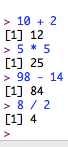It also provides functions such as sum() and sqrt() for addition and calculation of the square root. The following screenshot shows this in action: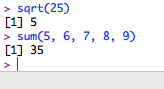R can—and will—do basic arithmetic like a calculator, using symbols you're familiar with. One you may not have used before is exponentiate, where you use two asterisks, for example, 4 ** 2, which you can read as 4 to the power of 2.

Once you want to start doing math beyond basic arithmetic, such as finding square roots or summing many numbers, you have to start using functions.

# Executing Basic Functions in the R Console

Let's now try and execute the sum() and sqrt() functions in R. Follow the steps given below:

1. Open the R console on your system.
2. Type the code as follows:
`sum(1, 2, 3, 4, 5)sqrt(144)`
1. Execute the code.

Output: The preceding code provides the following output: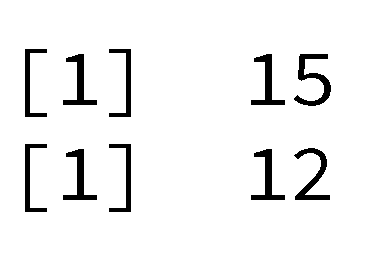Functions such as sum() and sqrt() are called base functions, which are built into R. This means that they come pre-installed when R is downloaded.

We could build all of our code right in the R console, but eventually, we might want to see our files, a list of everything in our global environment, and more, so instead, we'll use RStudio. Close R, and when it asks you to save the workspace image, for now, click Don't Save. (More explanation on workspace images and saving will come later in this chapter.)

Open RStudio. We'll use RStudio for all of our code development from here on. One major benefit of RStudio is that we can use Projects, which will organize all of the files for analysis in one folder on our computer automatically. Projects will keep all of the parts of your analysis organized in one space in a chosen folder on your machine. Any time you start a new project, you should start a new project in RStudio by going to File | New Project, as shown in the below screenshot, or by clicking the new project button (blue, with a green plus sign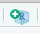).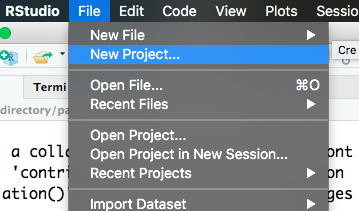Creating a project from a New Directory allows us to create a folder on our drive (here E:\) to store all code files, data, and anything else associated with the book. If there was an existing folder on our drive that we'd like to make the directory for the project, we would choose the Existing Directory option. The Version Control option allows you to clone a repository from GitHub or another version control site. It makes a copy of the project stored on GitHub and saves it on your computer: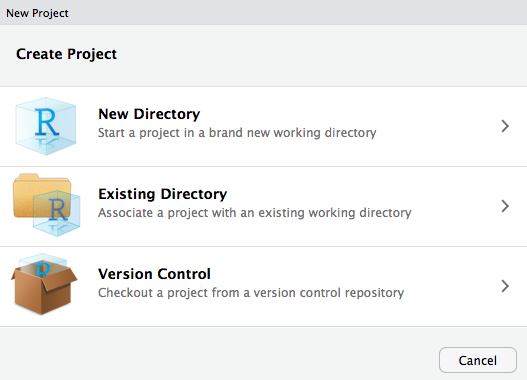The working directory in R is the folder where all of the code files and output will be saved. It should be the same as the folder you choose when you create a project from a new or existing directory. To verify the working directory at any time, use the getwd() function. It will print the working directory as a character string (you can tell because it has quotation marks around it). The working directory can be changed at any time by using the following syntax:

`setwd("new location/on the/computer")`

To create a new script in R, navigate to File | New File | R Script, as shown in the screenshot below, or click the button on the top left that looks like a piece of paper with a green arrow on it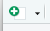.Inside New File, there are options to create quite a few different things that you might use in R. We'll be creating R scripts throughout this book.

Custom functions are fairly straightforward to create in R. Generally, they should be created using the following syntax:

`name_of_function <- function(input1, input2){operation to be performed with the inputs}`

The example custom function is as follows:

`area_triangle <- function(base, height){0.5 * base * height}`

Once the custom function code has been run, it will display in the Global Environment in the upper right corner and is now available for use in your RStudio project, as shown in the following screenshot: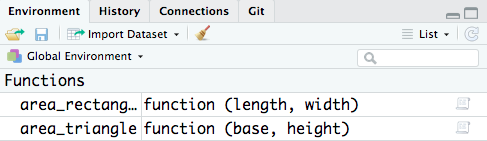One crucial step upon exiting RStudio or when you close your computer is to save a copy of the global environment. This will save any data or custom functions in the environment for later use and is done with the save.image() function, into which you'll need to enter a character string of what you want the saved file to be called, for example, introDSwR.RData. It will always be named with the extension .RData. If you were to open your project again some other time and want to load the saved environment, you use the load() function, with the same character string inside it.

# Setting up a New Project

Let us now set up a new project that we will use throughout the book. We will create an R project, script, custom function, and save an image of the global environment. Follow the steps given below:

1. Open RStudio.
2. Navigate to File | New Project to start a new project:
• Start with a new directory and save it in a place on your computer that makes sense to you.
• Save the project with the name IntroToDSwRCourse.
3. Check the working directory using the getwd() function and be sure it's the same folder you chose to save your project in.
4. Start a new script. Save the script with the filename lesson1_exercise.R.
5. Write a custom function, area_rectangle(), which calculates the area of a rectangle, with the following code:
`area_rectangle <- function(length, width){length * width}`
1. Try out area_rectangle() with the following sets of lengths and widths:
• 5, 10
• 80, 7
• 48209302930, 4

The code will be as follows:

`area_rectangle(5, 10)area_rectangle(80, 70)area_rectangle(48209302930, 4)`
1. Save an image of the global environment for later; name the file introToDSwR.RData.

Output: The output you get after executing the getwd() function will be the folder on your computer that you have chosen to save your project in.

The area of the rectangle with different lengths will be provided as follows: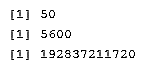R and RStudio will be our main tools throughout this book for statistical analysis and programming. We've now seen how to create a new project, a new R script, and how to save a workspace image for use later.

# Installing Packages

In this chapter, we've already seen some of the base functions that are built into R. We also built a few custom functions, area_triangle() and area_rectangle(), in the last section.

Now, let's talk about packages. Anyone can write an R package of useful functions and publish it for use by others. Packages are usually made available on the Comprehensive R Archive Network (CRAN) website, or Bioconductor, a collection of packages for bioinformatics and other uses. Both these sites conduct a thorough review of submitted packages before publishing them on their sites, so package developers often keep beta versions of packages, or those that are useful but may not pass the inspections, on GitHub.

RStudio makes installing packages very easy. There are two ways to do so: either type install.packages("package_name") in a script or your console, or you can navigate to the Packages tab in the lower right window and click Install, which will show a window that allows you to type the names of the packages that are available on CRAN. You can even install multiple packages if you separate them with a comma. You'll want to keep Install dependencies checked, as this will install any packages that the package you've chosen depends on to run successfully—you'll want those! Let's see this in the following screenshot: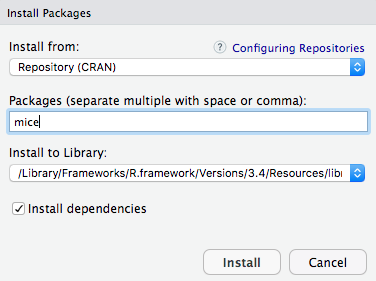Let's now use two different methods to install R packages. Follow these steps:

• Install the survival package using the following code:
`install.packages("survival")`
• Install the mice package using the Install button on the Packages tab.

Output: The following should be displayed on your console: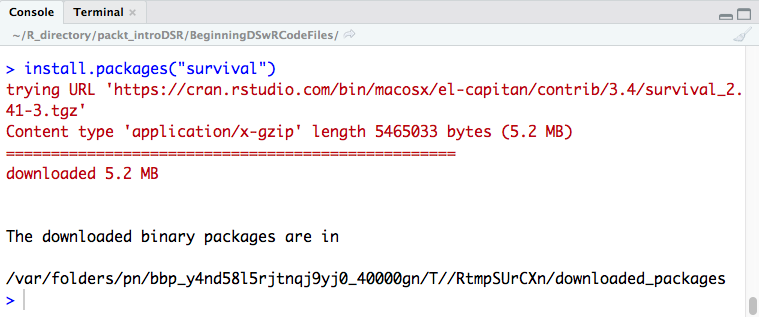The same information should be displayed once you install mice, with that package's name subbed in for survival.

R and RStudio, plus its packages, are incredibly helpful data science tools that will be the focus of this book. Now, let's get your project for the book set up, and install a set of incredibly useful packages called the Tidyverse, for use throughout the rest of this book.

# Activity: Installing the Tidyverse Packages

Scenario

You have been assigned the task of developing a report using R. You need to install the Tidyverse packages to develop that report.

Aim

To install Tidyverse, a set of useful packages that will be used later in the book. Load the inbuilt datasets into the project.

Prerequisites

Make sure that you have R and RStudio installed on your machine.

Steps for completion

1. Install the Tidyverse package using the install.package() function.
1. Load the ggplot2 library and the built-in msleep dataset.
Note that msleep is a built-in dataset in the ggplot2 package. We'll use R built-in datasets throughout this book.
1. Save the global image of the environment for use later use.

This activity was crucial. We've added a dataset and the Tidyverse packages to the project we intend to use for the rest of the book. We've also saved a copy of our global environment to our working directory. The Tidyverse packages, dplyr, ggplot2, tidyr, and a few others, will be very helpful throughout this book and in your data science work.

# Variable Types and Data Structures

In this section, we'll begin first with an exploration of different variable types: numeric, character, and dates. We'll then look at different data structures in R: vectors, lists, matrices, and data frames.

# Variable Types

Variable types exist in all programming languages and will tell the computer how to store and access a variable.

First, know that all variables created in R will have a class and a type. You can look at the class or type of anything in R using the class() and typeof() functions, respectively.

The class of an object is a broad designation, for example, character, numeric, integer, and date. These are very broad categories, and type elaborates more specifically on what type of variable it is, for example, a variable of class date can be of type character or POSIXct, depending on how it is stored. Type drills down into the details of a variable and how it's been stored in R, though sometimes class and type can be the same. For example, integers are of class and type integer, and character strings are of type and class character. Let's examine the following code snippet:

`x <- 4.2class(x)typeof(x)`

The preceding code provides the following output: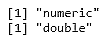In this snippet, x has a class numeric, because it is a number, but also has a type double because it is a decimal number. This is because all numeric data in R is of type double unless the object has been explicitly declared to be an integer. Let's look at some examples of different classes and types.

# Numeric and Integers

The numeric data class includes all numbers except integers, which are their own separate class in R. Anything of class numeric will be of type double, unless it is explicitly declared as an integer. To create an integer, you must type a capital letter, L, after a whole number.

Let's now create and check the class() and typeof() of different numeric objects in R. Follow the steps given below:

1. Create the following numeric objects:
`x <- 12.7y <- 8Lz <- 950`
1. Check the class and type of each using class() and typeof(), respectively, as follows:
`class(x)typeof(x)class(y)typeof(y)class(z)typeof(z)`

Output: The preceding code provides the following output: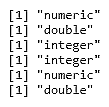# Character

Character data is always mentioned in quotation marks; anything contained in quotation marks is called a character string. Usually, character data is of both class and type character.

Let's create and check the class() and typeof() of different character objects in R. Follow the steps given below:

1. Create the following objects:
`a <- "apple"b <- "7"c <- "9-5-2016"`
1. Check the class and type of each using class() and typeof(), respectively, as follows:
`class(a)typeof(a)class(b)typeof(b)class(c)typeof(c)`

Output: The preceding code provides the following output: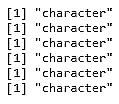# Dates

Dates are a special type of data in R, and are distinct from the date types POSIXct and POSIXlt, which represent calendar dates and times in more formal ways.

Let's create and check the class() and typeof() of different date objects in R. Follow these steps:

1. Create the objects using the following code:
`e <- as.Date("2016-09-05")f <- as.POSIXct("2018-04-05")`
1. Check the class and type of each by using class() and typeof(), respectively, as follows:
`class(e)typeof(e)class(f)typeof(f)`

Output: The preceding code provides the following output: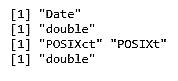One nice thing about R is that we can change objects from one type to another using the as.*() function family. If we have a variable, var, which currently has the value of 5, but as a character string, we can cast it to numeric data type using as.numeric() and to an integer using as.integer(), which the following code demonstrates:

`#char to numeric, integervar <- "5"var_num <- as.numeric(var)class(var_num)typeof(var_num)var_int <- as.integer(var)class(var_int)typeof(var_int).`

Conversely, we can go the other way and cast the var_num and var_int variables back to the character data type using as.character(). The following code demonstrates this:

`#numeric, integer to charvar <- 5#numeric to charvar_char <- as.character(var_num)class(var_char)typeof(var_char)#int to charvar_char2 <- as.character(var_int)class(var_char2)typeof(var_char2)`

A character string can be converted into a Date, but it does need to be in the format Year-Month-Day (Y-M-D) so that you can use the as.Date() function, as shown in the following code:

`#char to datedate <- "18-03-29"Date <- as.Date(date)class(Date)typeof(Date)`

There are formatting requirements for dates for them to save correctly. For example, the following code will not work:

`date2 <- as.Date("03-29-18")`

It will throw the following error:

`Error in charToDate(x) : character string is not in a standard unambiguous format`

It will be important to understand variable types throughout a data science project. It would be very difficult to both clean and summarize data if you're unsure of its type. In Chapter 3, Data Management, we'll also introduce another variable type: factors.

# Activity: Identifying Variable Classes and Types

Scenario

You need to write some code for classifying the data correctly for easy report generation. The following is the provided data:

 Variable Class Type "John Smith" 16 10L 3.92 -10 "03-28-02" as.Date("02-03-28")

Aim

To identify different classes and types of data in R.

Prerequisites

A pencil or pen, plus RStudio and R installed on your machine.

Steps for completion

1. Fill in the table provided with the class and type of each variable.
2. Use the class() or typeof() functions if you get stuck, but first try and fill it in without the code!

# Data Structures

There are a few different data structures in R that are crucial to understand, as they directly pertain to the use of data! These include vectors, matrices, and dataframes. We'll discuss how to tell the difference between all of these, along with how to create and manipulate them.

Data structures are extremely important in R for manipulating, exploring, and analyzing data. There are a few key structures that will hold the different types of variables we discussed in the last subsection, and more. R uses different words for some of these data structures than other programming languages, but the idea behind them is the same.

# Vectors

A vector is an object that holds a collection of various data elements in R, though they are limited because everything inside of a vector must all belong to the same variable type. You can create a vector using the method c(), for example:

` vector_example <- c(1, 2, 3, 4)`

In the above snippet, the method c() creates a vector named vector_example. It will have a length of four and be of class numeric. You use c() to create any type of vector by inputting a comma-separated list of the items you'd like inside the vector. If you input different classes of objects inside the vector (such as numeric and character strings), it will default to one of them.

In the following code, we can see an example where the class of the vector is a character because of the B in position 2:

`vector_example_2 <- c(1, "B", 3)class(vector_example_2)`

Output: The preceding code provides the following output: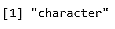To access a certain item in the vector, you can use indexing. R is a 1-indexed language, meaning all counting starts at 1 (versus other languages, such as Python, which are 0-indexed—that is, in Python, the first element of the array is said to be at the 0th position).

The first item in a vector can be accessed using vector, and so on. If the index doesn't exist in the vector, R will simply output an NA, which is R's default way of indicating a missing value. We'll cover missing values in R at length in Chapter 3, Data Management.

Let us now use c() to create a vector, examine its class and type, and access different elements of the vector using vector indexing. Follow the steps given below:

1. Create the vectors twenty and alphabet using the following code:
`twenty <- c(1:20)alphabet <- c(letters)`
1. Check the class and type of twenty and alphabet using class() and typeof(), respectively, as follows:
`class(twenty)typeof(twenty)class(alphabet)typeof(alphabet)`
1. Find the numbers at the following positions in twenty using vector indexing:
`twentytwentytwenty`
1. Find the letters at the following positions in the alphabet using vector indexing:
`alphabetalphabetalphabet`

Output: The code we write will be as follows:

`twenty <- c(1:20)alphabet <- c(letters)class(twenty)typeof(twenty)class(alphabet)typeof(alphabet)twentytwentytwentyalphabetalphabetalphabet`

The output we get after executing it is as follows: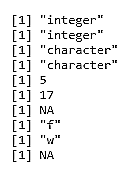# Lists

A list is different from a vector because it can hold many different types of R objects inside it, including other lists. If you have experience programming in another language, you may be familiar with lists, but if not, don't worry! You can create a list in R using the list() function, as shown in the following example:

`L1 <- list(1, "2", "Hello", "cat", 12, list(1, 2, 3))`

Let's walk through the elements of this list. First, we have the number 1. Then, a character string, "2", followed by the character string "Hello", the character string "cat", the number 12, and then a nested list, which contains the numbers 1, 2, and 3.

Accessing these different parts of the list that we just created is slightly different—now, you are using list indexing, which means using double square brackets to look at the different items.

You'll need to enter L1[] to view the number 1 and L1[] to see "cat".

To get inside the nested list, you'll have to use L1[] to see the number 1. L1[] gets us to the nested list, located at position 6, and L1[] allows us to access the first element of the nested list, in this case, number 1. The following screenshot shows the output of this code: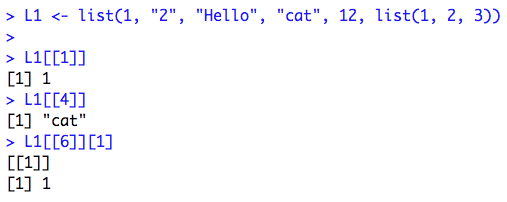Lists can also be changed into other data structures. We could turn a list into a dataframe, but this particular list, because it contains a nested list, will not coerce to a vector. The following code demonstrates this:

`L1_df <- as.data.frame(L1)class(L1_df)L1_vec <- as.vector(L1)class(L1_vec)`

The following screenshot shows the output of this code: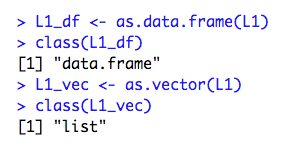# Matrices

A matrix is a 2D vector with rows and columns. In R, one requirement for matrices is that every data element stored inside it be of the same type (all character, all numeric, and so on). This allows you to perform arithmetic operations with matrices, if, for example, you have two that are both numeric.

Let's use matrix() to create a matrix, examine its class, use rownames() and colnames() to set row and column names, and access different elements of the matrix using multiple methods. Follow the steps given below:

1. Use matrix() to create matrix1, a 3 x 3 matrix containing the numbers 1:12 by column, using the following code:
`matrix1 <- matrix(c(1:12), nrow = 3, ncol = 3, byrow = FALSE)`
1. Create matrix2 similarly, also 3 x 3, and fill it with 1:12 by row, using the following code:
`matrix2 <- matrix(c(1:12), nrow = 3, ncol = 3, byrow = TRUE) `
1. Set the row and column names of matrix1 with the following:
`rownames(matrix1) <- c("one", "two", "three") colnames(matrix1) <- c("one", "two", "three")`
1. Find the elements at the following positions in matrix1 using matrix indexing:
`matrix1[1, 2]matrix1["one",]matrix1[,"one"]matrix1["one","one"]`

The output of the code is as follows: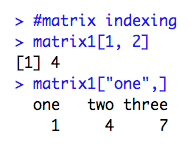# Dataframes

A dataframe in R is a 2D object where the columns can contain data of different classes and types. This is very useful for practical data storage.

Dataframes can be created by using as.data.frame() on applicable objects or by column- or row-binding vectors using cbind.data.frame() or rbind.data.frame(). Here's an example where we can create a list of nested lists and turn it into a data frame:

`list_for_df <- list(list(1:3), list(4:6), list(7:9))example_df <- as.data.frame(list_for_df)`

example_df will have three rows and three columns. We can set the column names just as we did with the matrix, though it isn't common practice in R to set the row names for most analyses. It can be demonstrated by the following code:

`colnames(example_df) <- c("one", "two", "three")`

We have covered a few of the key data structures in R in this section, and we have seen how to create and manipulate them. Let's try a few examples.

# Activity: Creating Vectors, Lists, Matrices, and Dataframes

Scenario

You have been asked to create vectors, lists, matrices, and dataframes that store information about yourself. The expected output is as follows: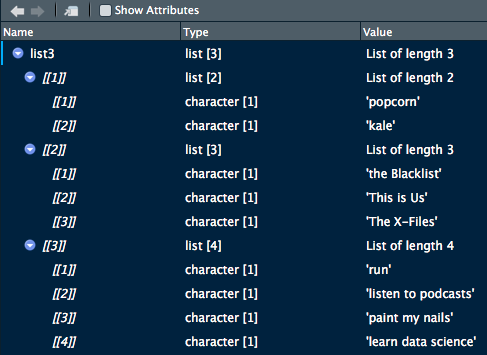Aim

To create vectors, lists, matrices, and dataframes.

Prerequisites

Make sure that you have R and RStudio installed on your machine.

Steps for Completion

1. Open a new R script and save it as a file called lesson1_activityB2.R.

2. Create vectors for the following:

• The numbers 1:10

• The letters A:Z, with the first four numbers and letters alternating

Hint: type ?LETTERS into your console.
1. Create lists for the following:
• The numbers 1:10
• The letters A:Z
• A list of lists:
• Your favorite foods (two or more)
• Your favorite TV shows (three or more)
• Things you like to do (four or more)
2. Create matrices of numbers and letters by using the following steps:
1. First, try using cbind() to combine the vector 1:10 and the vector A:Z. What happens?
2. Figure out a way to combine these two into a matrix, albeit one that will be coerced to character type (despite the numeric column).
3. Create dataframes using the following steps:
1. Coerce your matrix solution from the previous second bullet point into a dataframe. View it and take note of the type of each variable.
2. Use rbind.data.frame() to build a data frame where the rows increase by five until 25, for example, 5, 10, 15, 20, 25.
3. View it and notice how ugly the column names are. Give it better names ("one" through "five") with the names() function.

# Basic Flow Control

Flow Control includes different kinds of loops that you can use in R, such as the if/else, for, and while loops. While many of the concepts are very similar to how flow control and loops are used in other programming languages, they may be written differently in R.

Generally speaking, most loops are not considered best practice for coding in R. Some alternatives to loops, especially for loops, include the apply family of functions and functions contained in the purrr package, which you are encouraged to look up and learn about after this book.

# If/else

The if loop will only run a block of code if a certain condition is TRUE. It can be paired with else statements to create an if/else loop. This will work similarly to an if/else loop in other programming languages, though the syntax may be different.

The usual syntax for using if is as follows:

`if(test_condition){some_action}`

Here, the action only occurs if the test_condition evaluates to TRUE, so, for example, if you wrote 4 < 5, the code in the curly braces would definitely run.

If there's something you want to happen, even if the test condition isn't true, you would use an if/else, where the syntax usually looks like this:

`if(test_expression){some_action}else{some_other_action}`

Even if the test_expression isn't true, some_other_action will still happen. Finally, you can evaluate multiple test conditions with if/else if/else, as shown in the following syntax:

`if(test_expression){some_action}else if(another_test_expression){some_other_action}else{yet_another_action}`

Let's do some actual examples to help illustrate these points. Take a look at the following code:

`var <- "Hello"if(class(var) == "character"){print("Your variable is a character string.")}`

What output would you expect here? What output would you expect if the variable was assigned the value var <- 5 instead? When var is "Hello", the if statement is TRUE, and "Your variable is a character string" will print to the console. However, when var is 5, nothing happens, because we didn't specify an else statement.

With the following code, when var is 5, something will print:

`var <- 5if(class(var) == "character"){print("Your variable is a character string.")}else{print("Your variable is not a character")}`

Because we specified else, we will see the output "Your variable is not a character." This isn't very informative, however, so let's expand and use an else if:

`if(class(var) == "character"){print("Your variable is a character string.")}else if (class(var) == "numeric"){print("Your variable is numeric")}else{print("Your variable is something besides character or numeric.")}`

If var is 5, now we'll see "Your variable is numeric". What if var was a date? What would print then? Yup, you got it! "Your variable is something besides character or numeric" will print to the console.

# For loop

For loops are often used to go through every column or row of a dataframe in R.

Say, for example, that we're interested in the mean of all of the numeric columns of the built-in iris dataset (which is four out of the five—everything but the Species column, which is a factor variable of character strings indicating the species of each iris.) We could type, four times, mean(iris\$Sepal.Length), with each input variable name changing each time. However, a far more efficient way to complete this exercise would be to use a for loop.

If we simply want to print the means to the console, we could use a for loop as follows:

`for(i in seq_along(iris)){print(mean(iris[[i]]))}`

The output will be as follows: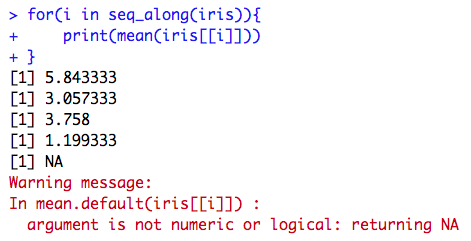We'll come back to the output, especially that warning message, in a second—first, let's break down the components of the for loop. The syntax will always be as follows:

`for(i in a range of numbers){some_action}`

In this particular for loop, we chose i as our iterator variable. A for loop in R will automatically iterate this variable, which means that every time it reaches the end of the loop, it will increase i by one. You might have noticed that once the loop has finished completing, i was added to the global environment as a Value, 5L (which means it's an integer, the number 5). Our iterator will always get added to the environment when a loop concludes.

It is displayed on the screen, as shown in the following screenshot: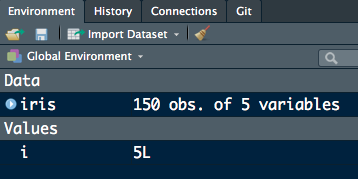The R function seq_along() is very helpful for the for loops, because it automatically moves along the number of columns of the dataframe (if that's the input) or more generally, iterates along the number of items contained in whatever is input into it.

We also chose to print the mean of each column in this particular for loop. Accessing the columns is done using indexing, so when i = 1, iris[[i]] is equal to the Sepal.Length variable, which is column 1, and so on. We got an error for column 5, because it isn't numeric (the Species variable!) Species doesn't have a mean, because it's a character variable.

This is actually a great example of where we can combine for loops with an if statement. Take a look at the following code:

`for(i in seq_along(iris)){    if(class(iris[[i]]) == "numeric"){        print(mean(iris[[i]]))    }}`

The if statement here will only print the mean of an iris column if the class of that column is numeric (which makes sense, since only numeric columns should have means!) The output is now only as follows: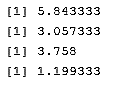If we're really feeling fancy, we could have even added an else statement with a different message for when the class of a column isn't numeric, such as in this loop:

`for(i in seq_along(iris)){    if(class(iris[[i]]) == "numeric"){        print(mean(iris[[i]]))    }else{        print(paste("Variable", i, "isn't numeric"))    }}`

The output is as follows: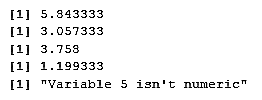seq_along() returns a sequence of numbers and makes for loops more straightforward. However, if you need to iterate using any other function, the syntax of the for statement will change slightly. The following code will print every row of the Species column in iris:

`for(i in 1:nrow(iris)){    print(iris[i, "Sepal.Width"])}`

You have to explicitly use 1:nrow(iris) in the for statement, or this loop will not run. nrow() simply returns the number of rows of iris versus the entire sequence of the number of columns that seq_along() returns as shown below:

`nrow(iris) 150seq_along(iris) 1 2 3 4 5`

# While loop

Versus the for loop, which walks through an iterator (usually, this is a sequence of numbers), a while loop will not iterate through a sequence of numbers for you. Instead, it requires you to add a line of code inside the body of the loop that increments or decrements your iterator, usually i. Generally, the syntax for a while loop is as follows:

`while(test_expression){some_action}`

Here, the action will only occur if the test_expression is TRUE. Otherwise, R will not enter the curly braces and run what's inside them. If a test expression is never TRUE, it's possible that a while loop may never run!

A classic example of a while loop is one that prints out numbers, such as the following:

`i = 0while(i <= 5){    print(paste("loop", i))    i = i + 1}`

The output of the preceding code is as follows: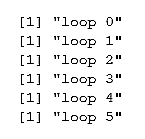Because we set our test expression to be i less than or equal to 5, the loop stopped printing once i was 6, and R broke out of the while loop. This is good, because infinite loops (loops that never stop running) are definitely possible. If the while loop test expression is never FALSE, the loop will never stop, as shown in the following code:

`while(TRUE){print("yes!")}`

The output will be as follows:

` "yes!" "yes!" "yes!" "yes!"…… `

This is an example of an infinite loop. If you're concerned about them, R does have a break statement, which will jump out of the while loop, but you'll see the following error:

`Error: no loop for break/next, jumping to top level`

This is because break statements in R are meant more for breaking out of nested loops, where there is one inside another.

It's also possible (though you likely wouldn't code this on purpose, as it will be an error of some kind) for a while loop to never run. For example, if we forgot that i is in our global environment, and that it equals 5, the following loop will never run:

`while(i < 5){    print(paste(i, "is this number"))    i = i + 1}`

Let's now try and get a feel of how loops work in R. We will try to predict what the loop code will print. Follow the steps below:

1. Examine the following code snippet. Try to predict what the output will be:
`vec <- seq(1:10)for(num in seq_along(vec)){    if(num %% 2 == 0){        print(paste(num, "is even"))    } else{        print(paste(num, "is odd"))    }}`
1. Examine the following code snippet. Try to predict what the output will be:
`example <- data.frame(color = c("red", "blue", "green"), number = c(1, 2, 3))for(i in seq(nrow(example))){print(example[i,1])}`
1. Examine the following code snippet. Try to predict what the output will be:
`var <- 5while(var > 0){    print(var)    var = var - 1}`

Output: The output for the first step will be as follows: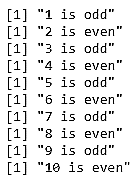The output for the second step will be as follows: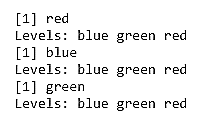The output for the third step will be as follows: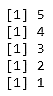It's important as you code in R that you understand how loops work, both because other people will write code with them and so you need to understand how other methods that can be substituted in for loops in R work.

# Activity: Building Basic Loops

Scenario

You've been asked to create loops to examine the variables inside the ChickWeight and iris built-in R datasets.

Aim

To implement of if, if/else, for, and while loops, including combinations of the four types of loops.

Prerequisites

You must have R and RStudio installed on your machine.

Steps for Completion

1. Open a new R script and save it with the name lesson1_activityC.R.
2. Load the iris and ChickWeight built-in R datasets. You will need to load them in separate data() functions.
3. If loop: Set var = 100 and create an if statement that prints Big number if var divided by five is greater than or equal to 25.
4. If/else: Expand and add an else statement that prints Not as big of a number
5. If/else if/else: In the middle, add an else if statement that prints Medium number if var divided by five is greater than or equal to 20.
6. For: Create a for loop that prints out Iris NUMBER is SPECIES for each row of iris:
Remember that seq_along() is for moving along columns. To move down rows, use seq(nrow(iris)). You may want to print the Species using an as.character() function, because it's a factor variable by default.
1. While: Set i = 12. While i > 0, print out i is a positive number, where i should be the number the loop is in.
1. For and if:
• For an extra challenge, first declare four NULL objects, Diet1 through Diet4.
• Use a for loop to loop through all the rows of ChickWeight. If the chick's diet is Diet1, add that row to Diet1 using the rbind() function. You should use rbind(Diet1, that row of ChickWeight).
• Then, check to see whether you got only the correct chicks in each dataset by viewing them.
This is by no means the best way of creating these four datasets, but it is an interesting challenge to think about how loops work.

# Data Import and Export

We've used some of R's built-in datasets so far in this book, but most of the time, data scientists will have to import and export data that comes from external sources in and out of R. Data can come and go in many different forms, and while we'll not cover them all here, we'll touch on some of the most common forms.

Data import and export are truly one subsection in R, because most of the time, the functions are opposites: for example, read.csv() takes in the character string name of a .csv file, and you save the output as a dataset in your environment, while write.csv() takes in the name of the dataset in your environment and the character string name of a file to write to.

There are built-in functions in R for the data import and export of many common file types, such as read.table() for .txt files, read.csv() for .csv files, and read.delim() for other types of delimited files (such as tab or | delimited, where | is the pipe operator appropriated as a separator). For pretty much any other file type, you have to use a package, usually one written for the import of that particular file type. Common data import packages for other types of data, such as SAS, Excel, SPSS, or Stata data, include the packages readr, haven, xlsx, Hmisc, and foreign: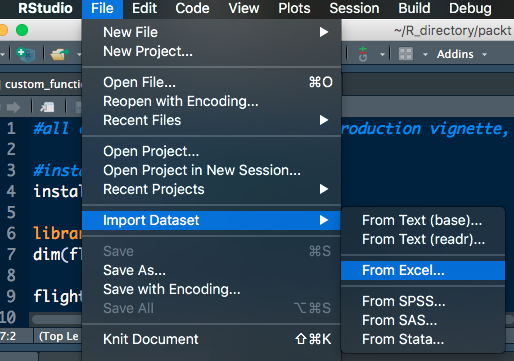RStudio also has point and click methods for importing data. If you navigate to File | Import Dataset, you'll see that you have six options:

• From text (base)
• From Excel
• From SPSS
• From SAS
• From Stata

These options call the required packages and functions that are necessary to input data of that type into R. The packages and functions are listed in order, as follows:

One advantage of loading data using one of these functions is that you can see the Import Text Data window. This window allows you to toggle different options, such as what to Name the dataset once it's imported, which Delimiter is used, how many rows to Skip, what value to use for missing data, and more. There's also a Code Preview, which allows you to see what the code will look like when you import your data. The following screenshot displays this: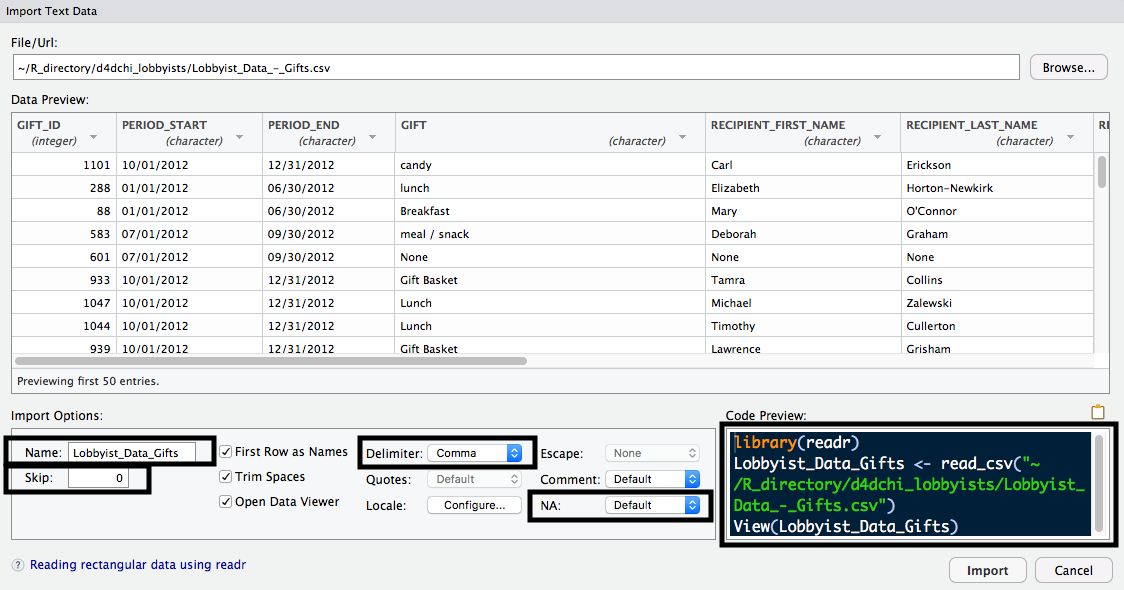To use most of these functions, you must follow these basic steps:

1. Figure out what type of data you're dealing with—is it a CSV? Is it from SAS? and so on.
2. Find the appropriate function to import that type of data.
3. The first (and sometimes only argument) to the function is a character string indicating where that data is located on your computer.
4. If applicable, tweak the settings of the appropriate function, such as indicating the separator with sep or setting stringAsFactors.
A synthetic dataset of 20 students, including their height in inches, weight in pounds, hair color, eye color, and United States' men's shoe sizes has been uploaded to the repository on GitHub, and can be found at the following URL: https://github.com/TrainingByPackt/R-Programming-Fundamentals/tree/master/lesson1.

Synthetic data means it was created for the purposes of this exercise and is not a dataset collected from an experiment. The dataset is saved in three formats:

• Text-delimited .txt file
• comma-separated values .csv file
• Microsof Excel worksheet .xlsx file

We'll be downloading all three of these directly from GitHub and importing them into RStudio. To do so, we'll need to use the URL, as a character string, as the first argument to all of the functions we'll use to import data.

When downloading data from GitHub directly, be sure you've clicked to view the Raw version of the dataset, as shown in the following screenshot: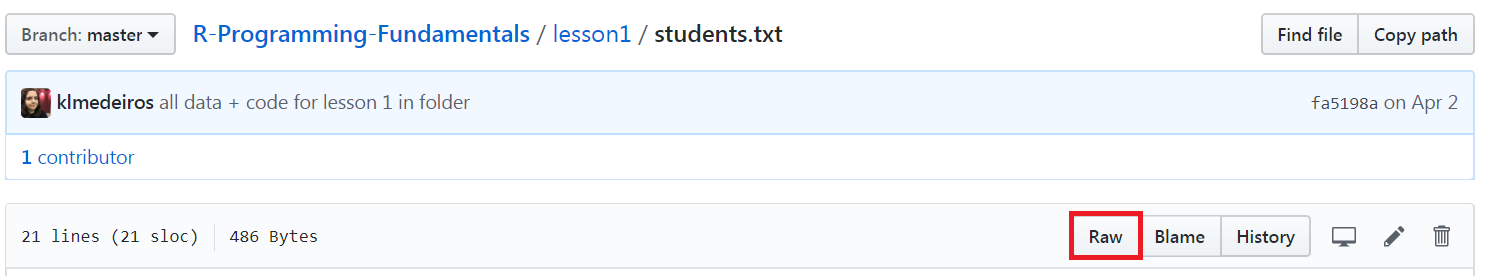We can import data directly from GitHub by using the read.table() function. If we input the URL where the dataset is stored, the function will download it for you, as shown in the following example:

`students_text <- read.table("https://raw.githubusercontent.com/TrainingByPackt/R-Programming-Fundamentals/master/lesson1/students.txt")`

While this code will read in the table, if we open and examine it, we will notice that the variable names are currently the first row of the dataset. To find the options for read.table(), we can use the following command:

`?read.table`

Reading the documentation, it says that the default value for the header argument is FALSE. If we set it to TRUE, read.table() will know that our table contains—as the first row—the variable names, and they should be read in as names. Here is the example:

`students_text <- read.table("https://raw.githubusercontent.com/TrainingByPackt/-Programming-Fundamentals/master/lesson1/students.txt",header = TRUE)`

The data has now been imported correctly, with the variable names in the correct place.

We may want to convert the Height_inches variable to centimeters and the Weight_ lbs variable to kilograms. We can do so with the following code:

`students_text\$Height_cm <- (students_text\$Height_inches * 2.54)students_text\$Weight_kg <- (students_text\$Weight_lbs / 0.453592)`

Since we've added these two variables, it may now be necessary to export the table out of R, perhaps to email it to a colleague for their use or to re-upload it on GitHub. The opposite of read.table() is write.table(). Take a look at the following example:

`write.table(students_text, "students_text_out.txt")`

This will write out the students_text dataset we're using in R to a file called students_text_out in our working directory folder on our machine.

There are additional options for read.table() that we could use as well. We can actually import a .csv file this way by setting the sep argument equal to a comma. We can skip rows with skip = some number, and add our own row names or column names by passing in a vector of either of those to the row.names or col.names argument.

Most of the other data import and export functions in R work much like read. table() and write.table(). Let's import the students' data from the .csv format, which can be done in three different ways, using read.table(), read.csv(), and read_csv().

1. Import the students.csv file from GitHub using read.table(). Save it as a dataset called students_csv1, using the following code:
`students_csv1 <-read.table("https://raw.githubusercontent.com/TrainingByPackt/R-Programming-Fundamentals/master/lesson1/students.csv", header = TRUE, sep = ",")`
1. Import students.csv using read.csv(), which works very similar to read. table():
`students_csv2 <- read.csv("https://raw.githubusercontent.com/TrainingByPackt/R-Programming-Fundamentals/master/lesson1/students.csv")`
`install.packages("readr")`
`library(readr)`
`students_csv3 <- read_csv("https://raw.githubusercontent.com/TrainingByPackt/R-Programming-Fundamentals/master/lesson1/students.csv")`
1. Examine students_csv2 and students_csv3 with str():
`str(students_csv2)str(students_csv3)`

Output:

The output for the str(students_csv2) function is as follows:

`'data.frame': 20 obs. of 5 variables:\$ Height_inches: int 65 55 60 61 62 66 69 54 57 58 …\$ Weight_lbs: int 120 135 166 154 189 200 250 122 101 178 …\$ EyeColor: Factor w/ 4 levels "Blue","Brown",…: 1 2 4 2 3 3 1 1 1 2 …\$ HairColor: Factor w/ 4 levels "Black","Blond",…: 3 2 1 3 2 4 4 3 3 1 …\$ USMensShoeSize: int 9 5 6 7 8 9 10 5 6 4 …`

The output for the str(students_csv3) function is as follows:

`Classes 'tbl_df', 'tbl' and 'data.frame': 20 obs. of 5 variables:\$ Height_inches : int 65 55 60 61 62 66 69 54 57 58 …\$ Weight_lbs : int 120 135 166 154 189 200 250 122 101 178 …\$ EyeColor : chr "Blue" "Brown" "Hazel" "Brown" … \$ HairColor : chr "Brown" "Blond" "Black" "Brown" …\$ USMensShoeSize: int 9 5 6 7 8 9 10 5 6 4 …`

A few notes about the three different ways we read in the .csv file in the exercise are as follows:

• For read.csv(), the header argument is TRUE by default, so we don't have to specify it:
• If we view the dataset, using str(students_csv2), we can see that the HairColor and EyeColor variables got read in as factor variables by default
• This is because the stringsAsFactors option is TRUE by default for read.csv()
• For read_csv() in the readr package, the opposite is true about the default stringsAsFactors value; it is now FALSE:
• Therefore, HairColor and EyeColor are read in as character variables. You can verify this with str(students_csv3)
• If we wanted factor variables, would have to change the variables ourselves after import or set stringsAsFactors = TRUE to automatically import all character variables as factors, as in read.csv()

One last common data type is Microsoft Excel spreadsheets, which are usually saved with the file extension .xlsx. We can use the xlsx R library, which contains read. xlsx() to import the students.xlsx file from GitHub. There's only one sheet in this file, but if there was more than one, we could specify which sheet to read in with the sheet argument.

Let's now use the xlsx package to import and export Microsoft Excel spreadsheet data. Follow the steps given below:

1. Navigate to the .xlsx version of the students dataset on GitHub, at the following link: https://github.com/TrainingByPackt/R-Programming-Fundamentals/blob/master/lesson1/students.xlsx.
4. Install and load the xlsx package by using the following code:
`install.packages("openxlsx")library(openxlsx)`
`students_xlsx <- read.xlsx("students.xlsx")`
1. Create a new variable in students_xlsx, called id, with the following code:
`students_xlsx\$id <- seq(1:nrow(students_xlsx))`
1. Export students_xlsx to your working directory:
`write.xlsx(students_xlsx, "students_xlsx_out.xlsx")`
1. Optionally, if you have a program installed on your machine that will open .xlsx files, open students_xlsx_out.xlsx and check to see whether the id variable exists.
If, instead of importing data directly from GitHub, you are looking to import data stored on your computer, do the following: Save the data in your working directory. Remember that this is the folder you've chosen to save your project in, which you can double check with getwd():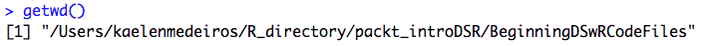1. As you import data, the first argument will be the name of the dataset as a character string (instead of the URLs we'll use throughout this chapter). For example, if students.txt was saved on your computer, you could import it with read_table("students.txt").

In your work as a data scientist, there are a few common data types you may encounter. The following table provides common data file types and explains how to import and export them in R:

These are, of course, not the only file types you can read in and out. R can read in data from everything listed, plus from other web datasets (a URL file), from SQL databases, JSON files, XML files, shapefiles for creating maps, and more. There are quite a few data types that R can use to handle importing, and usually a package is already written for doing so.

# Activity: Exporting and Importing the mtcars Dataset

Scenario

You have been asked to calculate a new variable in the mtcars built-in dataset and export the data so that you can email it to a colleague.

Aim

To export a dataset to a .csv file, edit it, and import it back into R using the appropriate functions.

Prerequisites

You must have R and RStudio installed on machine. Notepad, MS Excel, or any other program that can open .txt and .csv files will also be helpful.

Steps for Completion

1. Open a new R Script and save it as a file called lesson1_activityD.R.
2. Load the datasets library, and then the mtcars dataset.
3. Create a variable called hpcyl equal to the horsepower per cylinders of each car.
4. Write mtcars into a .csv file called mtcars_out.csv.
5. Read the dataset back in and call it mtcars_in by using read.csv().
Do you have to set the header or the stringsAsFactors argument? If you can't remember, check by typing ?read.csv into your console.

# Getting Help with R

We've covered a lot in this chapter, and this is the last thing that you'll carry throughout this book and into the rest of the time you spend learning R: how do you get help with R programming and data science?

# Package Documentation and Vignettes

One advantage of using R is that it is a very well-documented programming language. This is often because there is a certain amount of documentation that is required by CRAN before it will publish a package on the website.

It is considered a best practice to document your packages or functions well, no matter where you are publishing them. Good documentation is important, both for other people who may use your functions and also for yourself when you return to them in the future.

As such, there are a few built-in ways in R to get help. The first way to get help is to use the package documentation. You can access it by using the help() function or the question mark ?. These will do the same thing. For example, say you (this one happens a lot) can't remember off the top of your head the inputs to the glm() function. The following code will bring up the documentation for the glm() function, as shown in the following screenshot: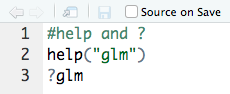If you read the documentation, you will see that you need to input at least a formula, family, and dataset name.

Of course, help() and the question mark ? can only help you with packages and functions you already know the name of. When you're not as sure, you can use help. search() and ?? to find things. These functions are also analogous, and will search the built-in help documentation for any and all instances of what you're looking for, for example, help.search("logit") or ??logit.

Both of the preceding options return a long list of help pages where logit appears. As you look through the results of your search for logit in the R documentation, you may notice that there are lots of things written in the format agricolae::reg.homog and base::Control.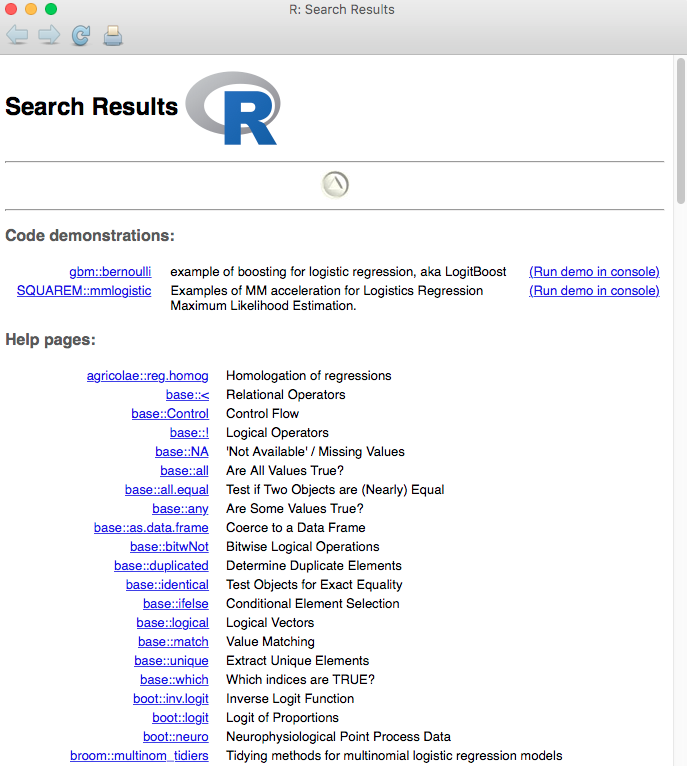In R, this notation means that the agricolae package has a function called reg.homog (agricolae::reg.homog), and that the base package has a function called Control (base::Control).

The double colon :: always separates a package and function name in R. (This comes in handy when you have functions named after the same thing in multiple packages, such as stats::filter and dplyr::filter!)

In addition to the often very helpful and thorough documentation built into R, some packages also have one or more vignettes, which are documents written by the author(s) of the package that are intended to demonstrate the main functionality of the functions contained in the package. You can bring these up inside RStudio in a number of ways.

Let's use the vignette-related functions browseVignettes() and vignettes() to explore the vignettes for R packages. Follow the steps given below:

1. To see a list of many available vignettes in R, use the browseVignettes() method:
• Browse the vignettes available for the dplyr package using the method syntax browseVignettes(package = "dplyr") or browseVignettes("dplyr")
2. Access the vignette for the tibble package using the syntax vignette("tibble") or vignette(package = "tibble").
3. In a search engine of your choice, find the vignette for the R package tidyr.

Check your Help tab to see the vignettes when you access them inside of RStudio. The output will be as follows: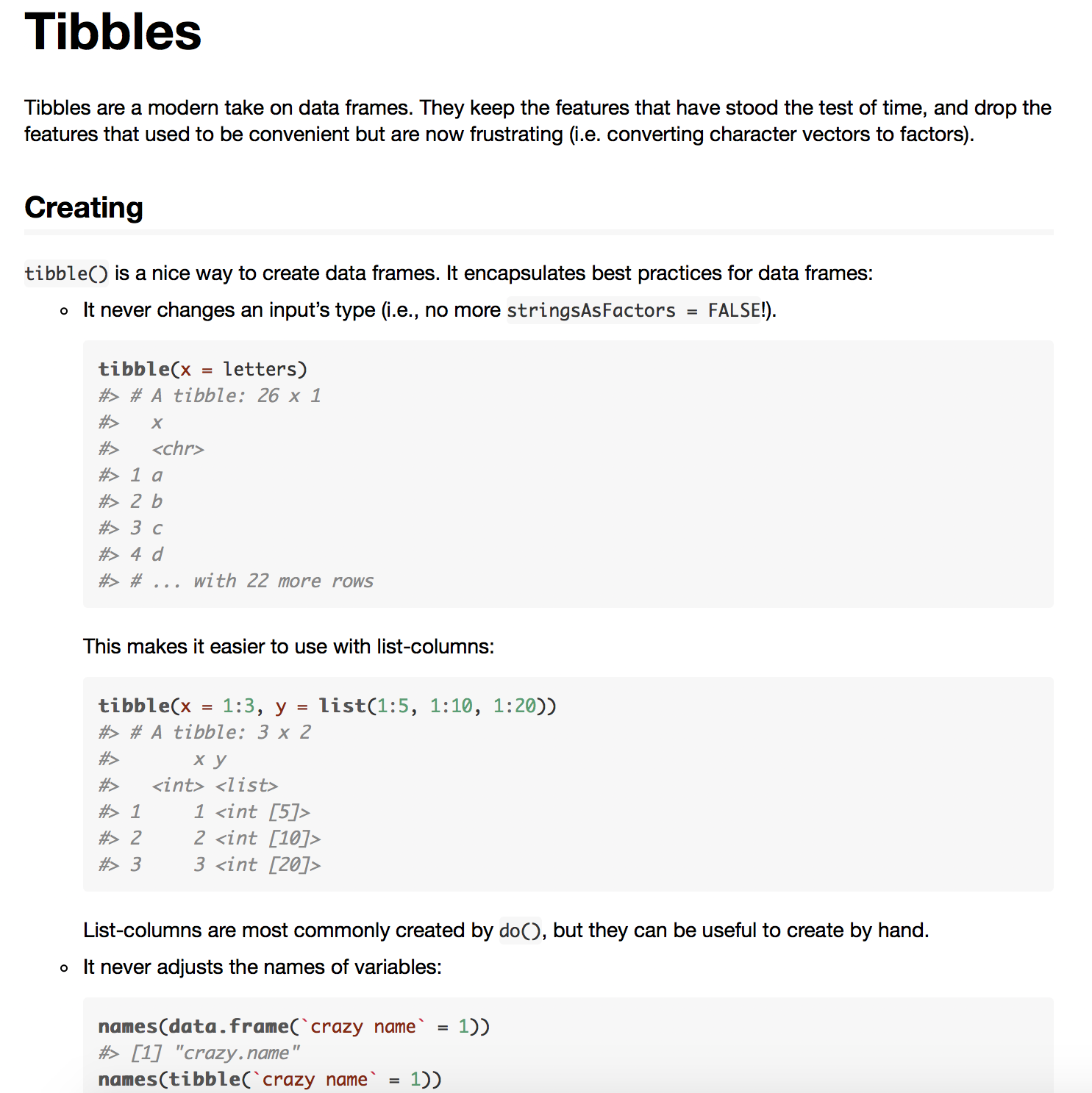# Activity: Exploring the Introduction to dplyr Vignette

Scenario

You have been asked to write code by utilizing the main verb functions (filter, arrange, select, mutate, and summarise) that are available in the dplyr package.

Aim

To gain experience of looking for vignettes and to also introduce the dplyr package.

Prerequisites

A web browser capable of looking at an R package vignette.

Steps for Completion

1. Navigate, in your web browser, to the introduction to the dplyr vignette (https://cran.r-project.org/web/packages/dplyr/vignettes/dplyr.html). dplyr is a package that is part of the Tidyverse we installed way back in the first section. You can also access the vignette inside of RStudio by running vignette("dplyr") in a script or by typing it in the console. It will appear in the Help tab in the lower right window.
2. Open up a new R Script (File | New File | R Script). Save it as a file called dplyr_vignette_walkthrough.R.
3. Read the dplyr vignette, running at least the first line of code for each of the main five dplyr verbs (filter, arrange, select, mutate, and summarise). Make sure that your output mirrors that of the vignette.
4. Stop when you reach the heading Patterns of operations, about halfway down the page. (It is recommended that you return later and read the entire vignette, as the dplyr package is very useful.)
5. If you have time, play with the dplyr code a bit and try to understand more about how the main five verbs work through experimentation.

# RStudio Community, Stack Overflow, and the Rest of the Web

Two of the main community-based resources online are the official RStudio Community and Stack Overflow.

Stack Overflow (SO) https://stackoverflow.com/ is a fantastic resource for a variety of questions relating to technology, with no limit on the different kinds of programming languages or analysis types you can ask questions about—the sky's the limit! There is an r tag (https://stackoverflow.com/questions/tagged/r) that has, as of late March 2018, 230,000+ tagged questions in it. Often, the best way to find what you're looking for is to go to a search engine, type something along the lines of how to relevel a factor in r, and often a SO post (or even multiple!) will be the top hits.

The RStudio Community (https://community.rstudio.com/) website is a forum that's run by RStudio themselves. It is expected that questions will be R-focused. They are often answered by the very people who wrote the packages and functions you are asking questions about. Questions are tagged by category, such as RMarkdown, General, and tidyverse, to help you navigate. The forum is searchable and filterable, and is a great place to get answers to any R-related questions.

More generally, #rstats on twitter (https://twitter.com/search?q=%23rstats&src=typd) is a great place to go for R questions, tips and tricks, and to find a community of people who are all over the R usage spectrum, from beginners to seasoned pros, who have often developed the packages used in R every day. Many R experts check the #rstats hashtag for questions, so it's another great way to get answers to R and data science queries. It's also a great way to find blog posts about R, which often include worked out examples that someone has solved, which are often useful as you are learning.

As learners new to R, you're sure to have many questions as you move on in your journey. Hopefully, you now know about and are even beginning to get comfortable with the many places both built into R and on the internet that you can go to for help, and eventually even help others as you gain confidence and skills.

# Summary

We've covered a lot in this introductory chapter and have plenty more to do, but don't fret! We'll continue to use plenty of examples and activities throughout to help you remember what we're learning. Let's press forward on to the next chapter, where we'll begin to look at some data more closely and do some cleaning and data management, which is necessary to get us one step closer to modeling and analysis.